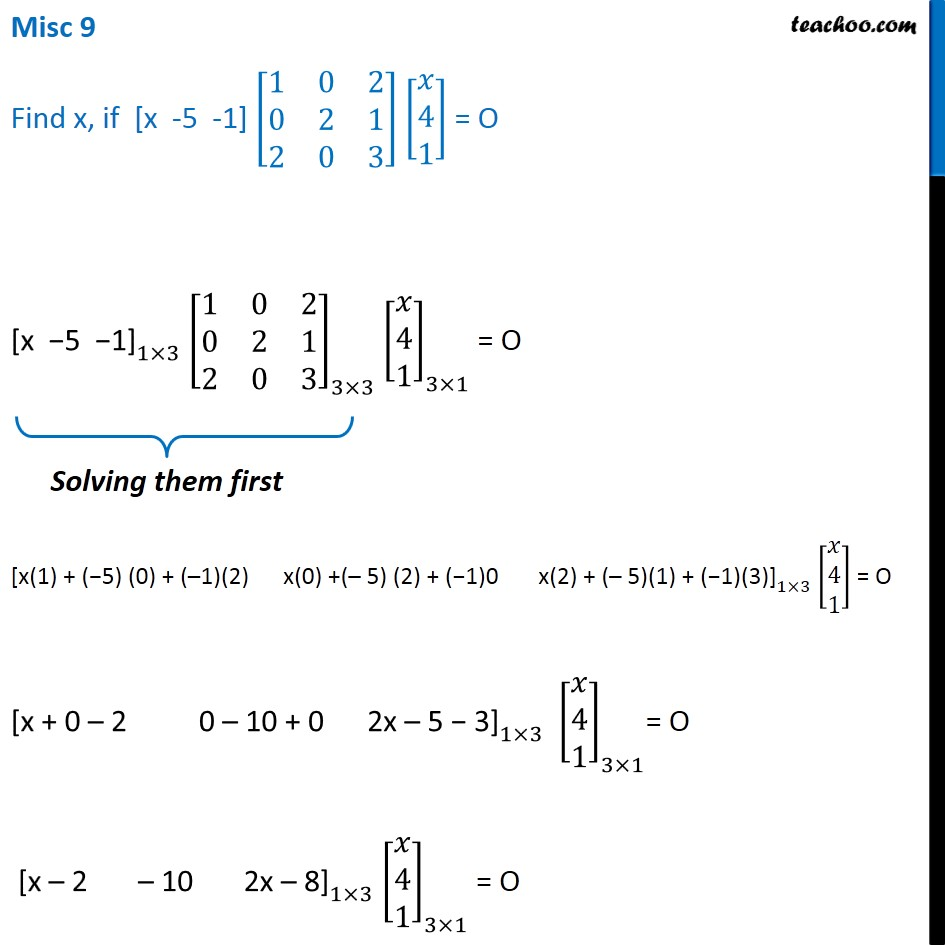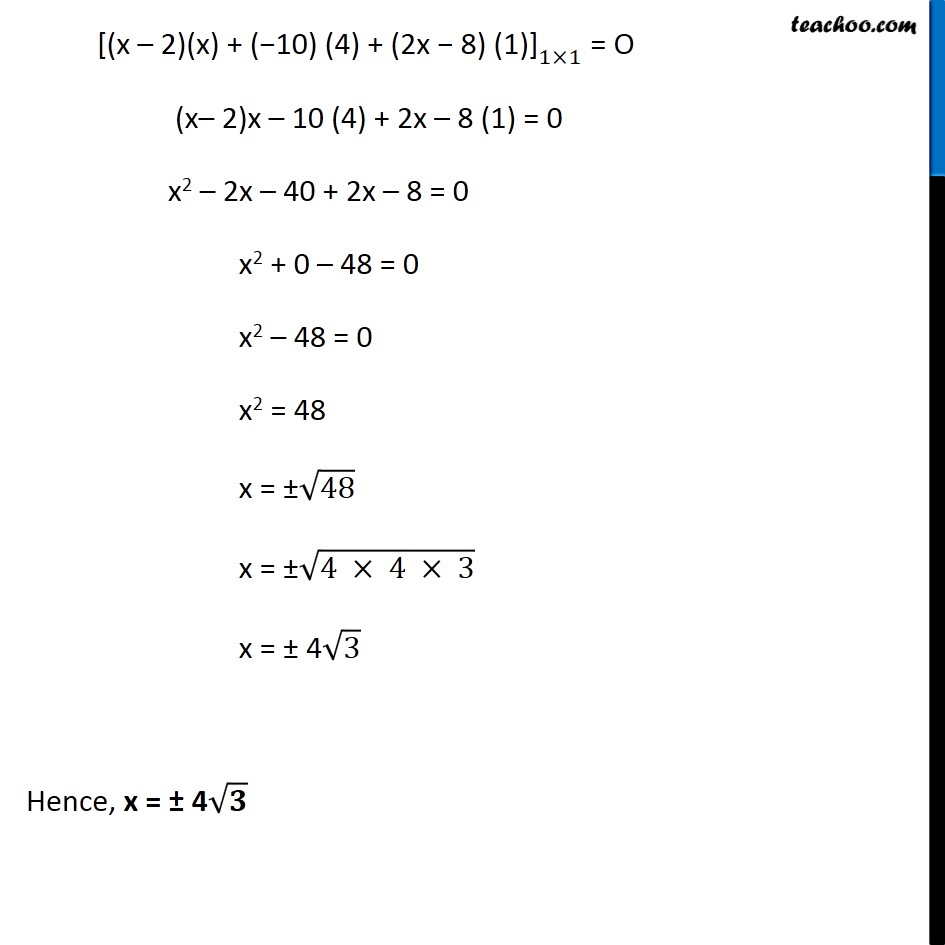Finding unknown - Element

Chapter 3 Class 12 Matrices
Concept wiseIntroducing your new favourite teacher - Teachoo Black, at only ₹83 per month

### Transcript

Misc 9 Find x, if [x -5 -1] [■8(1&0&2@0&2&1@2&0&3)] [■8(𝑥@4@1)] = O 〖"[x −5 −1]" 〗_(1×3) [■8(1&0&2@0&2&1@2&0&3)]_(3×3) [■8(𝑥@4@1)]_(3×1) = O 〖"[x(1) + (−5) (0) + (–1)(2) x(0) +(– 5) (2) + (−1)0 x(2) + (– 5)(1) + (−1)(3)]" 〗_(1×3) [■8(𝑥@4@1)] = O 〖"[x + 0 – 2 0 – 10 + 0 2x – 5 − 3]" 〗_(1×3) [■8(𝑥@4@1)]_(3×1)= O 〖"[x – 2 – 10 2x – 8]" 〗_(1×3) [■8(𝑥@4@1)]_(3×1) = O 〖"[(x – 2)(x) + (−10) (4) + (2x − 8) (1)]" 〗_(1×1) = O (x– 2)x – 10 (4) + 2x – 8 (1) = 0 x2 – 2x – 40 + 2x – 8 = 0 x2 + 0 – 48 = 0 x2 – 48 = 0 x2 = 48 x = ±√48 x = ±√(4 × 4 × 3) x = ± 4√3 Hence, x = ± 4√𝟑